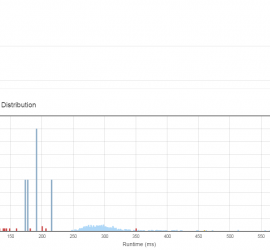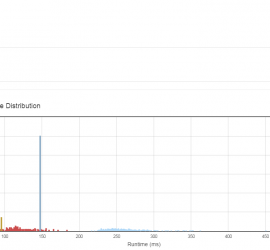# Daily Archives: March 29, 2015

## [leetcode] Path Sum II

Path Sum II Given a binary tree and a sum, find all root-to-leaf paths where each path’s sum equals the given sum. For example: Given the below binary tree and sum = 22, 5 / \ 4 8 / / \ 11 13 4 / \ / \ 7 2 […]## [leetcode] Jump Game II

Jump Game II Given an array of non-negative integers, you are initially positioned at the first index of the array. Each element in the array represents your maximum jump length at that position. Your goal is to reach the last index in the minimum number of jumps. For example: Given […]